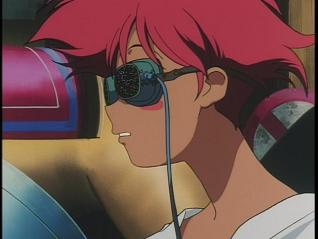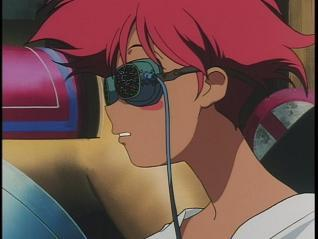OriginalAltered Image
filesize = 10115 bytes
width = 318
height = 239

<-- any number between -100 and 100.
<-- any number between -255 and 255. -255 is pure black and 255 is pure white.

Red = Green = Blue = <-- Any number between -255 and 255.
<-- The number of colors in the palette. Max is 25.
<-- any number between 1 and 255.
<-- any number between -8 and 8. ie -2 or 4.784.

<-- The number of colors in the palette. Max allowed is 25.
<-- any number between -360 and 360. Buggy